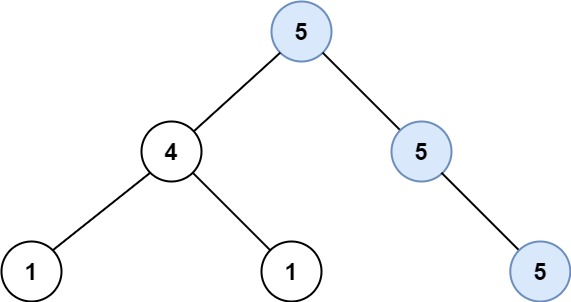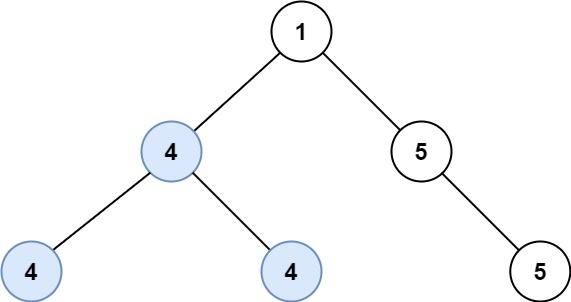# Longest Univalue Path - (Leetcode 687) - Coding Interview QuestionMani
Educating everyone with the beauty of programming!!

Given the `root` of a binary tree, return the length of the longest path, where each node in the path has the same value. This path may or may not pass through the root.

The length of the path between two nodes is represented by the number of edges between them.

Example 1:```Input: root = [5,4,5,1,1,5]
Output: 2
```

Example 2:```Input: root = [1,4,5,4,4,5]
Output: 2
```

Constraints:

• The number of nodes in the tree is in the range `[0, 104]`.
• `-1000 <= Node.val <= 1000`
• The depth of the tree will not exceed `1000`.

Solution:

``````1
2
3
4
5
6
7
8
9
10
11
12
13
14
15
16
17
18
19
20
21
22
23
24
25
26
27
28
29
30
31
32
33
34
35
36
37
38
39
/**
* Definition for a binary tree node.
* public class TreeNode {
* int val;
* TreeNode left;
* TreeNode right;
* TreeNode() {}
* TreeNode(int val) { this.val = val; }
* TreeNode(int val, TreeNode left, TreeNode right) {
* this.val = val;
* this.left = left;
* this.right = right;
* }
* }
*/
public class LongestUnivaluePath {
public int longestUnivaluePath(TreeNode root) {
if (root == null) {
return 0;
}
dfs(root, root.val);
return res - 1;
}

int res = 0;

int dfs(TreeNode root, int prev) {
if (root == null) {
return 0;
}
int left = dfs(root.left, root.val);
int right = dfs(root.right, root.val);
res = Math.max(res, 1 + left + right);
if (prev != root.val) {
return 0;
}
return 1 + Math.max(left, right);
}
}
``````
Drop your comments below for any questions or comments. For full list of must learn top interview questions Click Here: Must Learn Top Interview Questions

Rating: